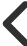Ideas Post### Sheeraz Ullah

@sheeraz# 15 ways to increase reading comprehension with ChatGPT

One thing I appreciate about ChatGPT is how it has helped me understand complex material. There are numerous approaches to comprehending information that are not dynamically baked into the content. Here are a few techniques I employ.

What are examples of mapping functions in the REACT framework?

Using an analogy, tell me about Generative AI

## 3. Explain it like I am five

Explain to me like I am five the concept of Markov chains?

## 4. Application and use cases

What are practical use cases for a Markov chain?

## 5. Compare and contrast

Is the Markov chain the same thing as Bayesian modelling?

## 6. Ask it to explain it in terms of something else you understand

The other day, I had trouble understanding the subset clause used to filter elements in Python. I am an expert in SQL, and I know SQL also does filtering. Maybe I could relate something I knew (SQL) with something I was learning (Python)

PROMPT
Can take this statement in Python and write it into an SQL statement:
tracks_sold = tracks_invoices.groupby(['tid','name']).agg({'quantity':sum})
RESPONSE
SELECT tid, name, SUM(quantity) AS quantity_sum
FROM tracks_invoices
GROUP BY tid, name

## 7. Explain first principles

Elon Musk also contemplated reducing the expenses involved in developing a more economical battery for a spaceship.

Using the First Principles, what is the most effective way to reduce the cost of the industrial-strength battery?

## 8. Learning plan using the Pareto Principle

Please give me a learning plan to understand large learning models. My time is limited, so using the Pareto principle, provides me with the 20% things I need to learn to become 80% effective.

## 9. Step by Step

Explain to me how this code works step by step

## 10. Ask what if questions or absurd questions (reductio ad absurdum)

What would happen if I changed the gravitational constant of the universe?

## 11. Personas and Perspective

Explain this to me as a programmer
Explain this to me as a psychologist
Explain this to me as an economist
Explain this to me as a philosopher

## 15. Deep Analysis

What are the alternatives to X, and why is X better?
What are the costs for X?
What are the opportunity costs for x?
Dependency: What are some underlying assumptions for X to be effective?
Causation: What conditions caused X to arise?
Past: What is the origin story for X?
Future: What is the future of X?
What are some potential black swans or unknown unknowns about X?
What are the risks of X?

0 Like.0 Commentchris407xand 5 more liked this0158400